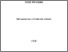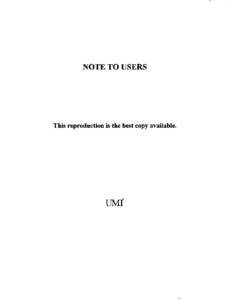Title:

# Decomposing and packing polygons / Dania el-Khechen.

el-Khechen, Dania (2009) Decomposing and packing polygons / Dania el-Khechen. PhD thesis, Concordia University.Preview
Text (application/pdf)
NR63446.pdf - Accepted Version
2MB

## Abstract

In this thesis, we study three different problems in the field of computational geometry: the partitioning of a simple polygon into two congruent components, the partitioning of squares and rectangles into equal area components while minimizing the perimeter of the cuts, and the packing of the maximum number of squares in an orthogonal polygon. To solve the first problem, we present three polynomial time algorithms which given a simple polygon P partitions it, if possible, into two congruent and possibly nonsimple components P 1 and P 2 : an O ( n 2 log n ) time algorithm for properly congruent components and an O ( n 3 ) time algorithm for mirror congruent components. In our analysis of the second problem, we experimentally find new bounds on the optimal partitions of squares and rectangles into equal area components. The visualization of the best determined solutions allows us to conjecture some characteristics of a class of optimal solutions. Finally, for the third problem, we present three linear time algorithms for packing the maximum number of unit squares in three subclasses of orthogonal polygons: the staircase polygons, the pyramids and Manhattan skyline polygons. We also study a special case of the problem where the given orthogonal polygon has vertices with integer coordinates and the squares to pack are (2 {604} 2) squares. We model the latter problem with a binary integer program and we develop a system that produces and visualizes optimal solutions. The observation of such solutions aided us in proving some characteristics of a class of optimal solutions.

Divisions: Concordia University > Gina Cody School of Engineering and Computer Science > Computer Science and Software Engineering Thesis (PhD) el-Khechen, Dania xiii, 158 leaves : ill. ; 29 cm. Concordia University Ph.D. Computer Science and Software Engineering 2009 Fevens, T 976664 Concordia University Library 22 Jan 2013 16:30 18 Jan 2018 17:43 http://clues.concordia.ca/search/c?SEARC...
All items in Spectrum are protected by copyright, with all rights reserved. The use of items is governed by Spectrum's terms of access.

Repository Staff Only: item control pageResearch related to the current document (at the CORE website)
Back to top